Home Practice
For learners and parents For teachers and schools
Textbooks
Full catalogue
Pricing SupportLog in

We think you are located in United States. Is this correct?

# 7.3 Measuring rates of reaction

## 7.3 Measuring rates of reaction (ESCN3)

How the average rate of a reaction is measured will depend on what the reaction is, what the reactants are, and what product forms. Look back at the reactions that have been discussed so far. In each case, how was the average rate of the reaction measured? The following examples will give you some ideas about other ways to measure the average rate of a reaction:

temp text

### Measuring the volume of gas produced per unit time (ESCN4)

The volume of gas produced in a reaction may be measured by collecting the gas in a gas syringe (Figure 7.4).

As more gas is produced, the plunger is pushed out and the volume of the gas in the syringe can be recorded.

By measuring the volume at set time intervals, we can graph the data (Figure 7.5) and hence determine the rate of the reaction.Figure 7.5: A plot of the volume of gas collected at set time intervals.

Examples of reactions that produce gas are listed below:

• Reactions that produce hydrogen gas:

When a metal reacts with an acid, hydrogen gas is produced. The hydrogen can be collected in a test tube. A lit splint can be used to test for hydrogen. The 'pop' sound shows that hydrogen is present.

For example, magnesium reacts with sulfuric acid to produce magnesium sulfate and hydrogen.

$\text{Mg}(\text{s}) + \text{H}_{2}\text{SO}_{4}(\text{aq}) \to \text{MgSO}_{4}(\text{aq}) + \text{H}_{2}(\text{g})$

• Reactions that produce carbon dioxide:

When a carbonate reacts with an acid, carbon dioxide gas is produced. When carbon dioxide is passed through limewater, it turns the limewater milky. A burning splint will also stop burning (be extinguished) in the presence of $$\text{CO}_{2}$$ gas. These are a simple tests for the presence of carbon dioxide.

For example, calcium carbonate reacts with hydrochloric acid to produce calcium chloride, water and carbon dioxide.

$\text{CaCO}_{3}(\text{s}) + 2\text{HCl}(\text{aq}) \to \text{CaCl}_{2}(\text{aq}) + \text{H}_{2}\text{O}(ℓ) + \text{CO}_{2}(\text{g})$

• Reactions that produce oxygen:

Hydrogen peroxide decomposes in the presence of a manganese(IV) oxide catalyst to produce oxygen and water.In the zinc and hydrochloric acid experiment the learners collect gas in a balloon. Do not light the gas in the balloon or allow it to be near flame. There will be an explosion.

## Measuring reaction rates

### Aim

To measure the effect of concentration on the average rate of a reaction.

### Apparatus

• Solid zinc granules, $$\text{1}$$ $$\text{mol.dm^{-3}}$$ hydrochloric acid $$(\text{HCl})$$

• Two conical flasks, two beakers, two balloons, bunsen burner, splint of wood

### Method

Do not get hydrochloric acid ($$\text{HCl}$$) on your hands. We suggest you use gloves and safety glasses whenever handling acids and handle with care.

1. Label a conical flask A. Weigh $$\text{5}$$ $$\text{g}$$ zinc granules into it.

Repeat with the second conical flask but label it B.2. Label a beaker $$\text{1}$$. Pour $$\text{10}$$ $$\text{cm^{3}}$$ $$\text{HCl}$$ into it.

Label the other beaker $$\text{2}$$. Pour $$\text{5}$$ $$\text{cm^{3}}$$ $$\text{H}_{2}\text{O}$$ into it. Add $$\text{5}$$ $$\text{cm^{3}}$$ $$\text{HCl}$$ to this second beaker.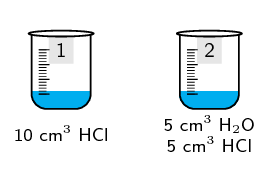3. Quickly: Pour the liquid in beaker $$\text{1}$$ into conical flask A and pour the liquid in beaker $$\text{2}$$ into conical flask B. Attach one balloon firmly to each conical flask.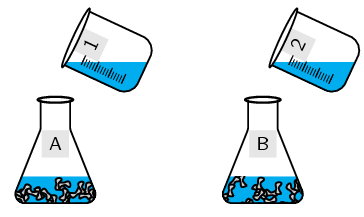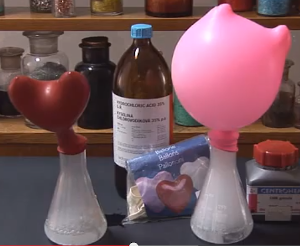4. Note which balloon filled more quickly.

5. Fill a test tube with the gas formed. Light only the gas in the test tube. Keep open flames away from the balloons.

The equation for this reaction is:

$$\text{Zn}(\text{s}) + 2\text{HCl}(\text{aq})$$ $$\to$$ $$\text{ZnCl}_{2}(\text{aq}) + \text{H}_{2}(\text{g})$$

### Results

• Which beaker contained the more concentrated solution of $$\text{HCl}$$?

• Which balloon filled more quickly?

• What happened when you lit the gas in the test tube?

### Conclusions

The more concentrated solution led to a faster reaction rate (i.e. the balloon filling with $$\text{H}_{2}$$ gas more quickly). The test for hydrogen gas would have resulted in a loud pop when the lit splint was placed near the mouth of a test tube.

### Precipitate reactions (ESCN5)

In reactions where a precipitate is formed, the amount of precipitate formed in a period of time can be used as a measure of the reaction rate. For example, when sodium thiosulfate reacts with an acid, a yellow precipitate of sulfur is formed. The reaction is as follows:

When the reaction between two liquids forms an insoluble compound, solid material will form in the liquid. This solid is called a precipitate.

$$\text{Na}_{2}\text{S}_{2}\text{O}_{3}(\text{aq}) + 2\text{HCl}(\text{aq})$$ $$\to$$ $$2\text{NaCl}(\text{aq}) + \text{SO}_{2}(\text{aq}) + \text{H}_{2}\text{O}(ℓ) + \text{S}(\text{s})$$

One way to estimate the average rate of this reaction is to carry out the investigation in a conical flask and to place a piece of paper with a black cross underneath the bottom of the flask. At the beginning of the reaction, the cross will be clearly visible when you look into the flask (Figure 7.6). However, as the reaction progresses and more precipitate is formed, the cross will gradually become less clear and will eventually disappear altogether. Measuring the time that it takes for this to happen will give an idea of the reaction rate. Note that it is not possible to collect the $$\text{SO}_{2}$$ gas that is produced in the reaction, because it is very soluble in water.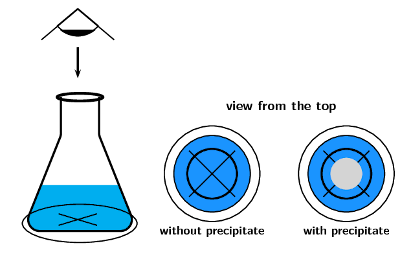Figure 7.6: At the beginning of the reaction between sodium thiosulfate and hydrochloric acid, when no precipitate has been formed, the cross at the bottom of the conical flask can be clearly seen. As the precipitate forms less of it can be seen.

For the reaction of sodium thiosulfate with hydrochloric acid it would be good to break your class up into groups and assign different experiments to them at this point. They can report back to the rest of the class.

This experiment is recommended for informal assessment in CAPS. Remember to remind the learners about laboratory safety procedures, especially when handling acids.

## Measuring reaction rates

### Aim

To measure the effect of concentration on the average rate of a reaction.

### Apparatus

• $$\text{300}$$ $$\text{cm^{3}}$$ of sodium thiosulfate $$\text{Na}_{2}\text{S}_{2}\text{O}_{3}$$ solution. (Prepare a solution of sodium thiosulfate by adding $$\text{12}$$ $$\text{g}$$ of $$\text{Na}_{2}\text{S}_{2}\text{O}_{3}$$ to $$\text{300}$$ $$\text{cm^{3}}$$ of water). This is solution 'A'.

• $$\text{300}$$ $$\text{cm^{3}}$$ of water

• $$\text{100}$$ $$\text{cm^{3}}$$ of $$\text{1}$$:$$\text{10}$$ dilute hydrochloric acid. This is solution 'B'.

• Six $$\text{100}$$ $$\text{cm^{3}}$$ glass beakers, measuring cylinders, paper and marking pen, stopwatch or timer

### Method

Do not get hydrochloric acid ($$\text{HCl}$$) on your hands. We suggest you use gloves and safety glasses whenever handling acids and that you handle with care.

One way to measure the average rate of this reaction is to place a piece of paper with a cross underneath the reaction beaker to see how long it takes until the cross cannot be seen due to the formation of the sulfur precipitate.

1. Set up six beakers on a flat surface and label them 1 to 6.

2. Pour $$\text{60}$$ $$\text{cm^{3}}$$ solution A into the first beaker and add $$\text{20}$$ $$\text{cm^{3}}$$ of water

3. Place the beaker on a piece of paper with a large black cross on it.

4. Use the measuring cylinder to measure $$\text{10}$$ $$\text{cm^{3}}$$ $$\text{HCl}$$. Now add this $$\text{HCl}$$ to the solution that is already in the first beaker (NB: Make sure that you always clean the measuring cylinder you have used before using it for another chemical).

5. Using a stopwatch with seconds, write down the time it takes for the precipitate that forms to block out the cross.

6. Now measure $$\text{50}$$ $$\text{cm^{3}}$$ of solution A into the second beaker and add $$\text{30}$$ $$\text{cm^{3}}$$ of water. Place the beaker over the black cross on the paper. To this second beaker, add $$\text{10}$$ $$\text{cm^{3}}$$ $$\text{HCl}$$, time the reaction and write down the results as you did before.

7. Continue the experiment by diluting solution A as shown below.

 Beaker Solution A ($$\text{cm^{3}}$$) Water ($$\text{cm^{3}}$$) Solution B ($$\text{cm^{3}}$$) Time (s) $$\text{1}$$ $$\text{60}$$ $$\text{20}$$ $$\text{10}$$ $$\text{2}$$ $$\text{50}$$ $$\text{30}$$ $$\text{10}$$ $$\text{3}$$ $$\text{40}$$ $$\text{40}$$ $$\text{10}$$ $$\text{4}$$ $$\text{30}$$ $$\text{50}$$ $$\text{10}$$ $$\text{5}$$ $$\text{20}$$ $$\text{60}$$ $$\text{10}$$ $$\text{6}$$ $$\text{10}$$ $$\text{70}$$ $$\text{10}$$

The equation for the reaction between sodium thiosulfate and hydrochloric acid is:

$$\text{Na}_{2}\text{S}_{2}\text{O}_{3}(\text{aq}) + 2\text{HCl}(\text{aq})$$ $$\to$$ $$2\text{NaCl}(\text{aq}) + \text{SO}_{2}(\text{aq}) + \text{H}_{2}\text{O}(ℓ) + \text{S}(\text{s})$$

### Results

• Calculate the reaction rate in each beaker. Remember that:

rate of the formation of product = $$\dfrac{ \text{moles product formed}}{\text{reaction time (s)}}$$

In this experiment you are stopping each experiment when the same approximate amount of precipitate is formed (the cross is blocked out by precipitate). So a relative reaction rate can be determined using the following equation:

reaction rate = $$\dfrac{1}{\text{time (s)}}$$

• Represent your results on a graph. Concentration will be on the x-axis and reaction rate on the y-axis. Note that the original volume of $$\text{Na}_{2}\text{S}_{2}\text{O}_{3}$$ can be used as a measure of concentration.

• Why was it important to keep the volume of $$\text{HCl}$$ constant?

• Describe the relationship between concentration and reaction rate.

### Conclusions

The higher the concentration of the reactants, the faster the average reaction rate.

### Changes in colour (ESCN6)

In some reactions there is a change in colour which tells us that the reaction is occuring. The faster the colour change the faster the reaction rate.

For example, when ethanoic acid (acetic acid) is titrated with sodium hydroxide, an indicator such as phenolphthalein is added. The solution is clear in an acidic solution and changes to pink when the reaction is complete. If the concentration of the base were increased, the colour change would happen faster (after a smaller volume of base was added), showing that a higher concentration of base increased the reaction rate.

$\text{CH}_{3}\text{COOH}(\text{aq}) + \text{NaOH}(\text{aq}) \to \text{Na}^{+}(\text{aq}) + \text{CH}_{3}\text{COO}^{-}(\text{aq}) + \text{H}_{2}\text{O}(ℓ)$### Changes in mass (ESCN7)

For a reaction that produces gas, the mass of the reaction vessel can be measured over time. The mass loss indicates the amount of gas that has been produced and escaped from the reaction vessel (Figure 7.7).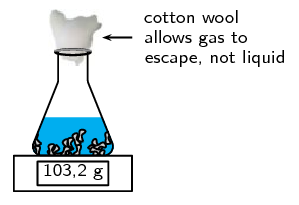Figure 7.7: A change in mass indicating the loss of a gas (photo by tjmwatson on Flickr).

If the reaction vessel is sealed this method will not work.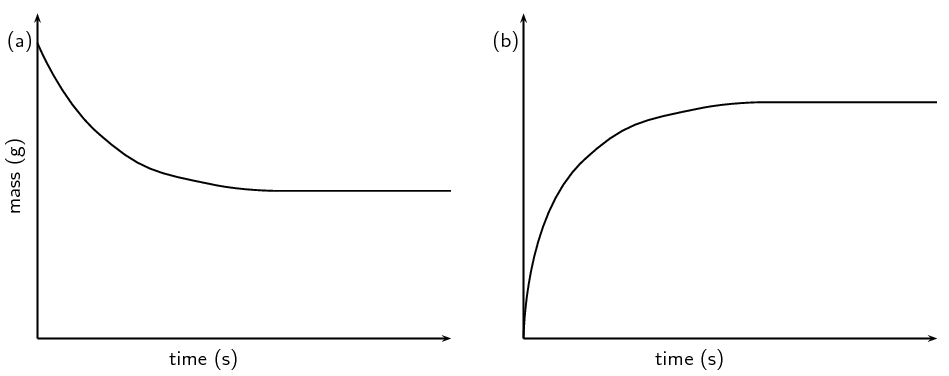Figure 7.8: a) A graph of the sample mass versus time and b) a graph of the mass loss versus time.

Notice the difference between the two graphs in Figure 7.8. The mass of the sample will decrease as the reaction proceeds. The mass lost from the sample will increase as the reaction proceeds. The material lost (that accounts for the mass loss) can be collected and measured (for example with the gas syringe method as shown in Figure 7.4).